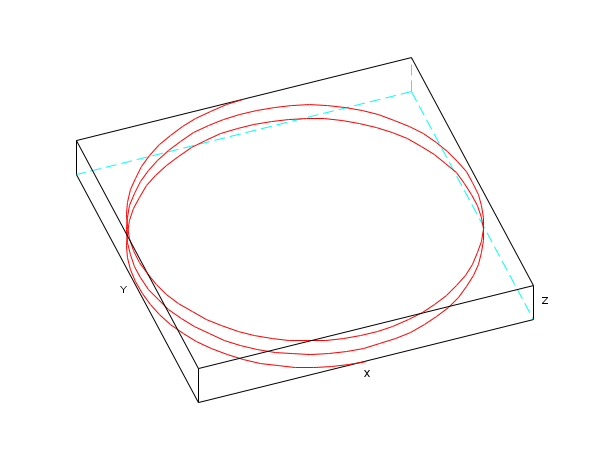Scilab Home page | Wiki | Bug tracker | Forge | Mailing list archives | ATOMS | File exchange
Please login or create an account
Change language to: English - Português - 日本語 - Русский

Please note that the recommended version of Scilab is 6.1.0. This page might be outdated.
See the recommended documentation of this function

Aide de Scilab >> Graphiques > 3d_plot > param3d

# param3d

3D plot of a parametric curve

### Syntax

`param3d(x,y,z,[theta,alpha,leg,flag,ebox])`

### Arguments

x,y,z

three vectors of the same size (points of the parametric curve).

theta, alpha

real values giving in degree the spherical coordinates of the observation point. The default values are 35 and 45 degree.

leg

string defining the labels for each axis with @ as a field separator, for example "X@Y@Z".

flag=[type,box]

`type` and `box` have the same meaning as in `plot3d`:

type

an integer (scaling).

type=0

the plot is made using the current 3D scaling (set by a previous call to `param3d`, `plot3d`, `contour` or `plot3d1`).

type=1

rescales automatically 3d boxes with extreme aspect ratios, the boundaries are specified by the value of the optional argument `ebox`.

type=2

rescales automatically 3d boxes with extreme aspect ratios, the boundaries are computed using the given data. This is the default value.

type=3

3d isometric with box bounds given by optional `ebox`, similarily to `type=1`.

type=4

3d isometric bounds derived from the data, similarily`to type=2`.

type=5

3d expanded isometric bounds with box bounds given by optional `ebox`, similarily to `type=1`.

type=6

3d expanded isometric bounds derived from the data, similarily to `type=2`.Note that axes boundaries can be customized through the axes entity properties (see axes_properties).

box

an integer (frame around the plot).

box=0

nothing is drawn around the plot.

box=1

unimplemented (like box=0).

box=2

only the axes behind the surface are drawn.

box=3

a box surrounding the surface is drawn and captions are added.

box=4

a box surrounding the surface is drawn, captions and axes are added.Note that axes aspect can also be customized through the axes entity properties (see axes_properties). This is the default value.

ebox

It specifies the boundaries of the plot as the vector `[xmin,xmax,ymin,ymax,zmin,zmax]`. This argument is used together with `type` in `flag` : if it is set to `1`, `3` or `5` (see above to see the corresponding behaviour). If `flag` is missing, `ebox` is not taken into account. Note that, when specified, the `ebox` argument acts on the `data_bounds` field that can also be reset through the axes entity properties (see axes_properties). The ebox default value is [0,1,0,1,0,1].

### Description

`param3d` is used to plot a 3D curve defined by its coordinates `x`, `y` and `z`. Note that data can also be got or modified through the surface entity properties (see surface_properties).

Note that properties like `rotation angles`, `colors` and `thickness` of the plotted curves can also be got or modified through the polyline entity properties (see polyline_properties).

Use `param3d1` to do multiple plots.

Enter the command `param3d()` to see a demo.

### Examples

```t=0:0.1:5*%pi;
param3d(sin(t),cos(t),t/10,35,45,"X@Y@Z",[2,3])

e=gce() //the handle on the 3D polyline

e.foreground=color('red');

a=gca(); //the handle on the axes
a.rotation_angles=[10 70];```### See also

• param3d1 — 3D plot of parametric curves
• plot3d — 3D plot of a surface

### Comments

Add a comment:
Please login to comment this page.

 Report an issue << nf3d 3d_plot param3d1 >>

 Scilab EnterprisesCopyright (c) 2011-2017 (Scilab Enterprises)Copyright (c) 1989-2012 (INRIA)Copyright (c) 1989-2007 (ENPC)with contributors Last updated:Tue Feb 14 15:06:37 CET 2017